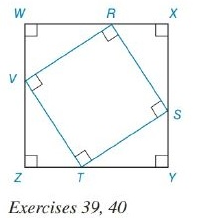Chapter 8.2, Problem 40EElementary Geometry For College St...

7th Edition
Alexander + 2 others
ISBN: 9781337614085

Solutions

Chapter
SectionElementary Geometry For College St...

7th Edition
Alexander + 2 others
ISBN: 9781337614085
Textbook Problem

Square RSTV is inscribed in square WXYZ, as shown. If Z T = 8 and T Y = 15 , Finda) the perimeter of RSTVb) the area of RSTVTo determine

To Find:

a. The perimeter of the square RSTV.

b. The area of the square RSTV.

Explanation

Formula Used:

1. Pythagorean theorem for the right angle triangle ABC for the hypotenuse AC,

AC2=AB2+BC2.

2. The perimeter of a polygon is the sum of the lengths of all sides of the polygon. P=4s, where s is side length of the square.

3. Area of the square =s2, where s is side length of the square.

It is given that the square RSTV is inscribed in square WXYZ with ZT=8 and TY=15.

The side ZY is split into ZT and TY with dimension 8 and 15 respectively.

As WXYZ is a square, each side of RSTV can also be split into two parts. That is, 8 and 15 respectively. Therefore, ZT=YS=XR=WV=8 and TY=SX=RW=VZ=15

Still sussing out bartleby?

Check out a sample textbook solution.

See a sample solution

The Solution to Your Study Problems

Bartleby provides explanations to thousands of textbook problems written by our experts, many with advanced degrees!

Get Started

Evaluate the integrals in Problems 1-26.

Mathematical Applications for the Management, Life, and Social Sciences

In Exercises 107-120, factor each expression completely. 116. (x + y)2 1

Applied Calculus for the Managerial, Life, and Social Sciences: A Brief Approach

The acceleration for a particle whose position at time t is is:

Study Guide for Stewart's Multivariable Calculus, 8th

The polar form for the graph at the right is:

Study Guide for Stewart's Single Variable Calculus: Early Transcendentals, 8th